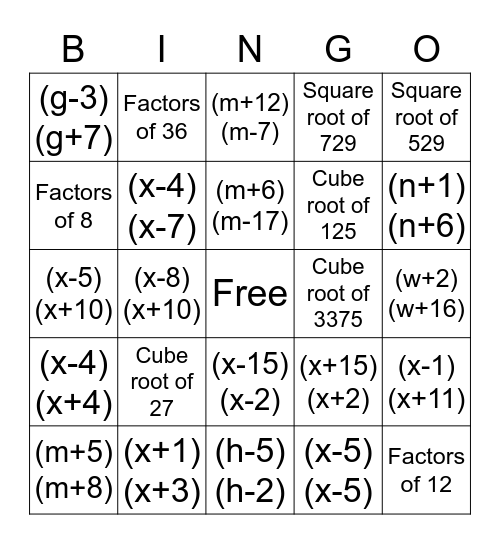# Factoring BingoThis bingo card has a free space and 34 words: (b+3)(b-5), (x-5)(x-5), (y+9)(y+3), (k+13)(k+1), (x-4)(x+4), (x-4)(x-7), Square root of 529, (m+5)(m+8), Factors of 36, (x+2)(x-7), (m+6)(m-17), (m+12)(m-7), (x+1)(x-8), (p+5)(p-5), Cube root of 3375, (n+1)(n+6), (x+1)(x+3), (x-5)(x+10), Cube root of 27, (x+15)(x+2), (x-15)(x-2), Factors of 18, (g-3)(g+7), (w+2)(w+16), (h-5)(h-2), Factors of 12, Square root of 729, Square root of 16, Cube root of 125, Factors of 8, Cube root of 8, (x-8)(x+10), (x-1)(x+11) and (x-9)(x+11).

## Play Online